## Measurement of Surface Tension of Liquid

### Experiment number : 2125

• #### Goal of experiment

The goal of this experiment is to show how to determine a surface tension of a liquid by dripping.

• #### Theory

Surface tension

Surface of a liquid behaves like a thin flexible membrane that tries to achieve an equilibrium state (state with minimum energy). With increasing surface, the energy of the surface layer increases. The surface of the liquid hence tries to take the smallest and smoothest area.

The value of surface tension decreases with increasing temperature.

Surface tension measurement

One of the methods to measure surface tension of a liquid is to let the liquid drip.

Liquid in a capillary tube forms drops at the bottom of the tube. The drops will gradually grow and, when they reach a sufficient size, they will separate from the liquid.

The gravitational force acts upon a drop with mass m:

$F_{\mathrm{g}} = mg.$

The drop is held at the end of the capillary tube by surface forces. The net force of these forces acts upwards and its magnitude is calculated from relationship

$F_{\mathrm{p}} = σl = σπd,$

where σ is the surface tension of the liquid, l is the circumference of the drop dripping from the capillary and d its diameter.

The drop will separate from the tube when the gravitational force is greater than the net force of the surface forces. Therefore:

$F_{\mathrm{p}} = F_{\mathrm{g}}$

After substituting from the equations above:

$σπd = mg.$

The surface tension is then calculated from the relationship

$σ = \frac{mg}{πd}.$

Using this formula, it would be possible to determine the surface tension. The accuracy of this measurement is negatively affected by the fact that only a part of the drop is separated from the tube when the drop drips; part of it stays on the end of the capillary. Before separating, the drop is narrowed and its diameter is smaller than d. Therefore, the dripping method is used mainly as a reference method. If we work with the same capillary, the diameter of the narrowed drop is approximately the same with all wetting liquids.

We use two different liquids in this experiment. We measure the surface tension σ1 of one liquid; we must know the surface tension σ2 of the second liquid (which can be looked up). We then determine the ratio of the surface tensions of these liquids

$\frac{σ_{1}}{σ_{2}} = \frac{\frac{m_{1}g}{πd}}{\frac{m_{2}g}{πd}} = \frac{m_{1}}{m_{2}}.$

First we determine the mass of one drop of each liquid (weigh more drops and divide the result by the number of drops). We substitute these masses m1 and m2 into the relationship

$σ_\mathrm{1} = \frac{m_\mathrm{1}}{m_\mathrm{2}}σ_\mathrm{2}.$

You can find the solved task here: Oil Pipetting.

• #### Tools

• liquid with unknown surface tension we want to measure

• liquid with surface tension we know (e.g. water)

• laboratory stand

• funnel

• cork with a hole (of a size to fit the capillary and the funnel firmly into it)

• capillary

• container into which we drop the measured liquid

• laboratory scales

• thermometer

Note: If you do not have these tools, you can use a capillary or pipette to drip the liquid (see Technical notes).

Fig 1: Tools for dripping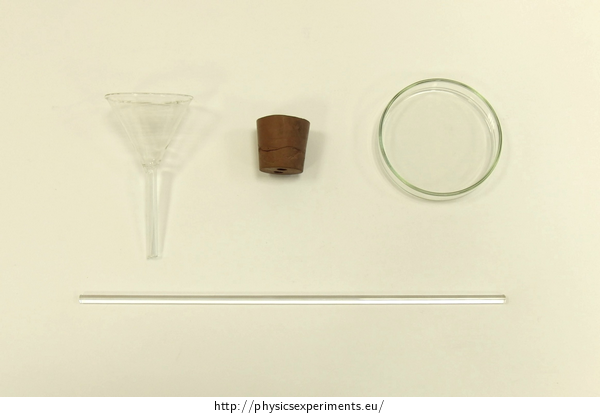• #### Procedure

1. Insert the funnel in one side of the hole in the cork and the capillary in the other. Fasten the cork in the laboratory stand.

2. Weigh the empty container which you later use to catch the drops.

3. Measure the temperature of both liquids.

4. Pour the liquid in the funnel.

5. Drip 100 drops (if we want to measure more accurately, we can drip more drops).

6. Weigh the container with 100 drops.

7. Determine the mass of 100 drops and then the mass of one drop.

8. Use the same procedure to determine the mass of one drop of water (second liquid).

9. Calculate the surface tension of the liquid from the relationship

$σ_\mathrm{1} = \frac{m_\mathrm{1}}{m_\mathrm{2}}σ_\mathrm{2}.$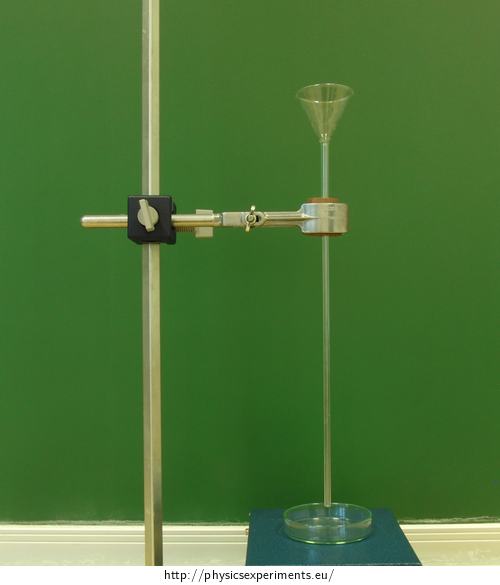• #### Sample result

We made the measurements with water, alcohol and kerosene. We weighed the container and let all the liquids drip into it.

The temperature of both liquids was 20 °C.

We used water as the reference liquid with known surface tension (σ1 = 72.75·10−3 N/m at temperature 20 °C).

Table 1 summarizes the mass of 100 drops of each liquid m100, the mass of one drop of each liquid m1 and the surface tension σ.

 - water alcohol kerosene m100 [g] 3.984 1.333 1.497 m1 [g] 3.98·10−2 1.33·10−2 1.50·10−2 σ [N·m−1] 72.75·10−3 23.95·10−3 27.42·10−3

For comparison, the table values for alcohol and kerosene at 20 °C (Czech source: Converter):

 alcohol 22.55·10−3 N/m kerosene 27.00·10−3 N/m
• #### Technical notes

We can use a pipette or a capillary to perform this experiment. Draw the measured liquid into the pipette or the capillary, block the upper end with your finger and let the liquid drip. We can influence the drip rate by pushing the top of the pipette.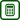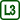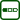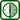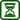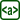×Original source: Bartuška, K., Svoboda, E.: Fyzika pro gymnázia. Molekulová fyzika a termika., Prometheus, Praha 2003